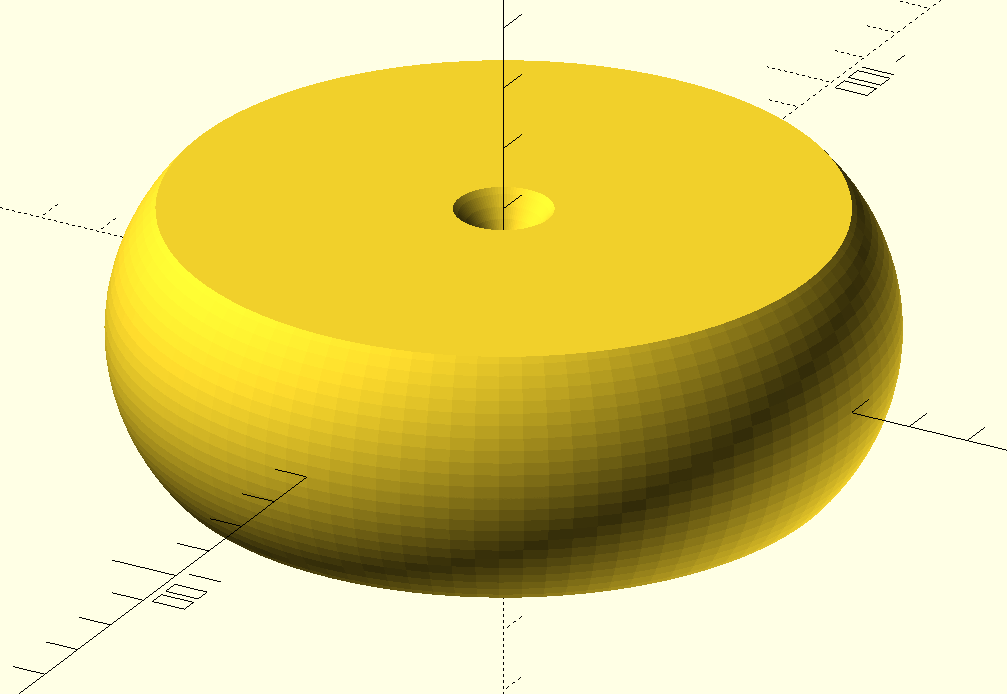### Error while rendering; what's wrong?R
richard@itrgmbh.de
Fri, Jan 14, 2022 1:24 PM

Hi all,

what’s wrong with my simple code?
Preview is generated successfully, but rendering fails with message:

Rendering Polygon Mesh using CGAL...

ERROR: The given mesh is not closed! Unable to convert to CGAL_Nef_Polyhedron.

My code:

``````module ring(d,b,r)
``````
``````{
``````
``````    intersection()
``````
``````    {
``````
``````        union(){
``````
``````            rotate_extrude(convexity = 10,\$fn = 100)
``````
``````                translate([d/2, 0, 0])
``````
``````                    circle(r = r,\$fn=100);
``````
``````        }
``````
``````        cylinder(d=d+r*2, h=b, center=true,\$fn = 100);
``````
``````    }
``````
``````}
``````

ring(60,40,30);

I am using current version: OpenSCAD Version: 2021.01 (under Windows)

Many thanks, Richard

Hi all, what’s wrong with my simple code?\ Preview is generated successfully, but rendering fails with message: ” Rendering Polygon Mesh using CGAL... ERROR: The given mesh is not closed! Unable to convert to CGAL_Nef_Polyhedron.  “ My code: ``` module ring(d,b,r) ``` ``` { ``` ``` intersection() ``` ``` { ``` ``` union(){ ``` ``` rotate_extrude(convexity = 10,\$fn = 100) ``` ``` translate([d/2, 0, 0]) ``` ``` circle(r = r,\$fn=100); ``` ``` } ``` ``` cylinder(d=d+r*2, h=b, center=true,\$fn = 100); ``` ``` } ``` ``` } ``` ring(60,40,30); I am using current version: **OpenSCAD Version:** 2021.01 (under Windows) Many thanks, RichardR
richard@itrgmbh.de
Fri, Jan 14, 2022 1:27 PM

p.s. Preview screenshot:G
Fri, Jan 14, 2022 1:43 PM

translate([60/2, 0, 0]) circle(r = 30,\$fn=100);
results in an object starting at 0. you cannot rotate_extrude that.

On Fri., Jan. 14, 2022, 08:28 , richard@itrgmbh.de wrote:

p.s. Preview screenshot: